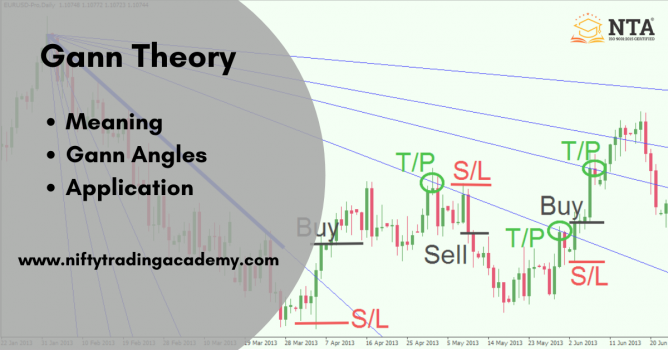Create an Account

Its Free and always will be!

Birthday
Day
Font size: +

# What is Gann Theory?

First let us start by describing the definition of Gann Theory:

## What is Gann Theory?

Gann theory predicts the momentum of stocks after taking into account the past, present and future of the markets.

By doing research and assessing the data’s of the different period including the short-term market highs and long-term market highs, angles are drawn to determine the future market trend.

Gann was a believer that mathematical relationships and geometrical angles can predict the future price movements.

The concept and theory of Gann is dependent on the angles, now let us observe about Gann theory.### What is Gann Angles?

• The Gann theory predicts the price movements with the help of series of angles.
• The angles are drawn at different price points.
• The Gann angles represent the past, present and future characteristics of a stock price.
• In fact, the calculation of Gann angles is done after connecting the price points on the charts.

Now let us talk about the application of Gann Theory:

### Application of Gann Theory:

To understand the Gann theory calculations one needs to study the behavior of the following three things in the market.

#1 Time Study: The time study model suggests the time when the stock price shall reverse. In addition, time study reads the historical data that helps in deriving the future fate of the markets.

#2 Price Study:The Gann angle let us see the angles suggesting the price patterns of the stock. The prediction of price movement in the stock relies on the pivot levels, support and resistance points.

#3 Pattern Study: The activity of the investors and movement particular stock is kept in check with pattern study. The pattern study in Gann theory studies the trends and patterns that indicate the reversal in the stock.

This was the concept about Gann Theory.

Rate this blog entry: